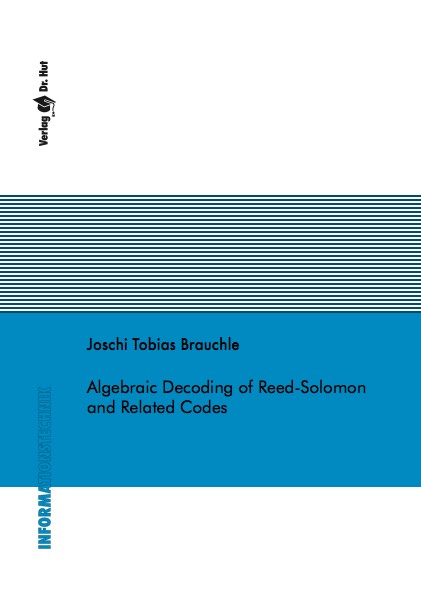Datenbestand vom 12. August 2022aktualisiert am 12. August 2022

# ISBN 978384392857172,00 € inkl. MwSt, zzgl. Versand

Joschi Tobias Brauchle
Algebraic Decoding of Reed-Solomon and Related Codes

127 Seiten, Dissertation Technische Universität München (2015), Softcover, A5

## Zusammenfassung / Abstract

This thesis addresses two topics of algebraic coding theory and is separated into two parts.

The first part of the work studies multivariate interpolation-based list-decoding (MID) of Reed-Solomon (RS) and related codes. We consider generalized and interleaved generalized RS codes, Parvaresh-Vardy codes and two versions of folded RS codes, all of which are decodable beyond half their minimum distance by using list-decoding. The construction and the properties of the aforementioned codes are presented and embedded into a universal code description. Linear-algebraic MID is introduced, then described and solved by linear systems of equations. The number of correctable errors, or decoding radius, of MID is derived in a general form for the universal code construction and specified explicitly for the codes under consideration. The size of the solution space, i.e., the output list size, is the main contributor to the computational complexity of linear-algebraic MID. We relate the size of the output list to the weight enumerator function of RS codes and use this relationship to quantify and bound its value. Numerical and simulation results on the codeword error rate, the probability of decoding failure and the complexity of linear-algebraic MID are presented.

The second part of this thesis studies generalized minimum distance (GMD) decoding, which allows making use of reliability information on the received symbols by applying an algebraic error-erasure decoder in a multi-trial manner. An algebraic decoder can correct more erasures than errors; the ratio between these numbers is called the error-erasure tradeoff of the decoder. In each decoding trial, a certain number of low reliability symbols are erased, and the modality of erasing these symbols is of critical importance for the success of the decoding procedure. We investigate different erasing strategies based on either erasing a fraction of the symbols or erasing all symbols below a certain reliability threshold, and these strategies may either be static or adapt to the received sequence. The optimal fraction or threshold and the position of the erasures is determined, and the maximum achievable decoding radius after a certain number of decoding trials is derived for all considered strategies. It is shown that the number of trials needed is significantly lower for decoders of low error-erasure tradeoff, which is the case for interpolation-based list-decoding considered in the first part of the work.